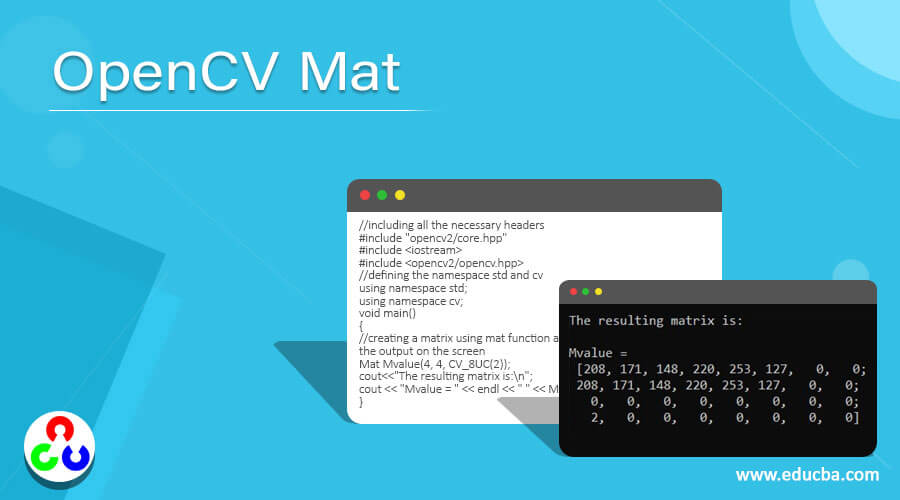Home Breaking OpenCV Mat | Working of Mat() Function in OpenCV | Examples

# OpenCV Mat | Working of Mat() Function in OpenCV | Examples## Introduction to OpenCV Mat

The images captured using cameras, scanners etc. capture the numerical values at each points of the image which are nothing but the values of the pixels at those points and in order to store and handle the images in the form of a matrix and to manage the memory associated with the images, we make use of class called Mat in OpenCV and by making use of Mat class in OpenCV, the memory management happens automatically and the memory allocated for a Mat class object can be reused and this class is available in the package opencv2/core.hpp in the OpenCV C++ library.

Syntax to define Mat() function in OpenCV:

`cv2::Mat::Mat(int rows, int columns, int type, const Scalar &s)`

Where,

• rows is the rows of the matrix.
• columns is the columns of the matrix.
• type is the data type used to store the elements in the matrix.
• Scalar &s specifies the value to be stored in the matrix.

### Working of Mat() Function in OpenCV

• Mat is a class in OpenCV consisting of two data parts namely matrix header and a pointer to the matrix.
• The information like size of the matrix, the method used for storing the matrix, the address at which the matrix must be stored etc. is available in the matrix header.
• The Mat function takes four parameters namely rows, columns, data type and scalar.
• The rows parameter passed to the Mat function represents the rows in a matrix.
• The columns parameter passed to the Mat function represents the columns in a matrix.
• The data type parameter passed to the Mat function represents the type of the elements stored in the matrix.
• The convention followed to specify the data type of the elements in the matrix is CV_[The number of bits per item][Signed or Unsigned][Type Prefix]C[The channel number].
• The scalar parameter specifies the data to be stored in the matrix.

### Examples of OpenCV Mat

Given below are the examples of OpenCV Mat:

#### Example #1

OpenCV program in C++ to create a matrix using Mat function and display the matrix as the output on the screen.

Code:

```//including all the necessary headers #include "opencv2/core.hpp" #include <iostream> #include <opencv2/opencv.hpp> //defining the namespace std and cv using namespace std; using namespace cv; void main() { //creating a matrix using mat function and displaying the matrix as the output on the screen Mat Mvalue(4, 4, CV_8UC3, Scalar(1, 0, 1)); cout<<"The resulting matrix is:n"; cout << "Mvalue = " << endl << " " << Mvalue << endl << endl; }```

Output:In the above program, we are including the necessary headers. Then we are defining the namespaces std and cv. Then the main method is defined within which we are creating a matrix using Mat function and displaying the matrix as the result on the screen.

#### Example #2

OpenCV program in C++ to create a matrix using Mat function and display the matrix as the output on the screen.

Code:

```//including all the necessary headers #include "opencv2/core.hpp" #include <iostream> #include <opencv2/opencv.hpp> //defining the namespace std and cv using namespace std; using namespace cv; void main() { //creating a matrix using mat function and displaying the matrix as the output on the screen Mat Mvalue(4, 4, CV_8UC(2)); cout<<"The resulting matrix is:n"; cout << "Mvalue = " << endl << " " << Mvalue << endl << endl; }```

Output:In the above program, we are including the necessary headers. Then we are defining the namespaces std and cv. Then the main method is defined within which we are creating a matrix using Mat function and displaying the matrix as the result on the screen.

#### Example #3

OpenCV program in C++ to create a matrix using Mat function and display the matrix as the output on the screen.

Code:

```//including all the necessary headers #include "opencv2/core.hpp" #include <iostream> #include <opencv2/opencv.hpp> //defining the namespace std and cv using namespace std; using namespace cv; void main() { //creating a matrix using mat function and displaying the matrix as the output on the screen Mat Mvalue(2, 2, CV_32F, Scalar(1,1,1)); cout<<"The resulting matrix is:n"; cout << "Mvalue = " << endl << " " << Mvalue << endl << endl; }```

Output:In the above program, we are including the necessary headers. Then we are defining the namespaces std and cv. Then the main method is defined within which we are creating a matrix using Mat function and displaying the matrix as the result on the screen.

#### Example #4

OpenCV program in C++ to create a matrix using Mat function and display the matrix as the output on the screen.

Code:

```//including all the necessary headers #include "opencv2/core.hpp" #include <iostream> #include <opencv2/opencv.hpp> //defining the namespace std and cv using namespace std; using namespace cv; void main() { //creating a matrix using mat function and displaying the matrix as the output on the screen Mat Mvalue(4, 4, CV_64F); cout<<”The resulting matrix is:n"; cout << "Mvalue = " << endl << " " << Mvalue << endl << endl; }```

Output:In the above program, we are including the necessary headers. Then we are defining the namespaces std and cv. Then the main method is defined within which we are creating a matrix using Mat function and displaying the matrix as the result on the screen.

### Recommended Articles

This is a guide to OpenCV Mat. Here we discuss the introduction, working of Mat() function in OpenCV and examples respectively. You may also have a look at the following articles to learn more –

The post OpenCV Mat appeared first on EDUCBA.

Source link